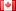# Revision history [back]

### Simplify, Solve Equation and use solution

Hi, I'm new to sage, and have the following question: I have the following code:

x,y=var('x y')

f(x,y)= sin(x)*cos(y)+sin(y)*cos(x)
f.full_simplify
show(f(x,y))

s1=solve([f(x,y)==1],x,y)
show(s1)


Sage obiously doesn't simplify the function f... (simple trigonometric expression). And it doesn't solve it.

1) How can I simplify the function? 2) How can I use the result? For example: I have as an result: solution=[x == -1] How can I replace this x in an other function (example: sin(x)). In mathematica I would do: sin(x) /. solution or manually: sin(x) /. x->(-1)

### Simplify, Solve Equation and use solution

Hi, I'm new to sage, and have the following question: I have the following code:

x,y=var('x y')

f(x,y)= sin(x)*cos(y)+sin(y)*cos(x)
f.full_simplify
show(f(x,y))

s1=solve([f(x,y)==1],x,y)
show(s1)


Sage obiously doesn't simplify the function f... (simple trigonometric expression). And it doesn't solve it.

1) 1) How can I simplify the function? 2) function?

2) How can I use the result? For example: I have as an result: solution=[x == -1] How can I replace this x in an other function (example: sin(x)). In mathematica I would do: sin(x) /. solution or manually: sin(x) /. x->(-1)3 No.3 RevisionDSM5212 ●21 ●68 ●111

### Simplify, Solve Equation and use solution

Hi, I'm new to sage, and have the following question: I have the following code:

x,y=var('x y')

f(x,y)= sin(x)*cos(y)+sin(y)*cos(x)
f.full_simplify
show(f(x,y))

s1=solve([f(x,y)==1],x,y)
show(s1)


Sage obiously doesn't simplify the function f... (simple trigonometric expression). And it doesn't solve it.

1) How can I simplify the function?

2) How can I use the result? For example: I have as an result: solution=[x == -1] How can I replace this x in an other function (example: sin(x)). In mathematica I would do: sin(x) /. solution or manually: sin(x) /. x->(-1)